# How to create external reference in Excel to refer to another sheet or workbook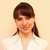by , updated on

This short tutorial explains the basics of an external reference in Excel, and shows how to reference another sheet and workbook in your formulas.

When calculating data in Excel, you may often find yourself in a situation when you need to pull data from another worksheet or even from a different Excel file. Can you do that? Of course, you can. You just need to create a link between the worksheets (within the same workbook or in different workbooks) by using what is called an external cell reference or a link.

External reference in Excel is a reference to a cell or a range of cells outside the current worksheet. The main benefit of using an Excel external reference is that whenever the referenced cell(s) in another worksheet changes, the value returned by the external cell reference is automatically updated.

Although external references in Excel are very similar to cell references, there are a few important differences. In this tutorial, we'll start with the basics and show how to create various external reference types with detailed steps, screenshots and formula examples.

## How to reference another sheet in Excel

To reference a cell or range of cells in another worksheet in the same workbook, put the worksheet name followed by an exclamation mark (!) before the cell address.

In other words, in an Excel reference to another worksheet, you use the following format:

Reference to an individual cell:

For example, to refer to cell A1 in Sheet2, you type Sheet2!A1.

Reference to a range of cells:

Sheet_name!First_cell:Last_cell

For example, to refer to cells A1:A10 in Sheet2, you type Sheet2!A1:A10.

Note. If the worksheet name includes spaces or non-alphabetical characters, you must enclose it in single quotation marks. For example, an external reference to cell A1 in a worksheet named Project Milestones should read as follows: 'Project Milestones'!A1.

In a real-life formula, which multiplies the value in cell A1 in 'Project Milestones' sheet by 10, an Excel sheet reference looks like this:

`='Project Milestones'!A1*10`

#### Creating a reference to another sheet in Excel

When writing a formula that refers to cells in another worksheet, you can of course type that other sheet name followed by an exclamation point and a cell reference manually, but this would be a slow and error-prone way.

A better way is point to the cell(s) in another sheet that you want the formula to refer to, and let Excel take care of the correct syntax of your sheet reference. To have Excel insert a reference to another sheet in your formula, do the following:

1. Start typing a formula either in a destination cell or in the formula bar.
2. When it comes to adding a reference to another worksheet, switch to that sheet and select a cell or a range of cells you want to refer to.
3. Finish typing the formula and press the Enter key to complete it.

For example, if you have a list of sales figures in sheet Sales and you want to calculate the Value Added Tax (19%) for each product in another sheet named VAT, proceed in the following way:

• Start typing the formula =19%* in cell B2 on sheet VAT.
• Switch to sheet Sales, and click on cell B2 there. Excel will immediately insert an external reference to that cell, as shown in the following screenshot: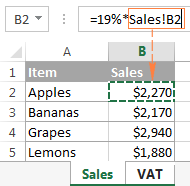• Press Enter to complete the formula.

Note. When adding an Excel reference to another sheet using the above method, by default Microsoft Excel adds a relative reference (with no \$ sign). So, in the above example, you can just copy the formula to other cells in column B on sheet VAT, the cell references will adjust for each row, and you will have VAT for each product correctly calculated.

In a similar manner, you can reference a range of cells in another sheet. The only difference is that you select multiple cells on the source worksheet. For example, to find out the total of sales in cells B2:B5 on sheet Sales, you would enter the following formula:

`=SUM(Sales!B2:B5)`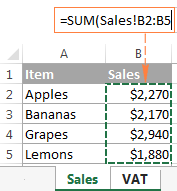This is how you reference another sheet in Excel. And now, let's see how you can refer to cells from a different workbook.

## How to reference another workbook in Excel

In Microsoft Excel formulas, external references to another workbook are displayed in two ways, depending on whether the source workbook is open or closed.

#### External reference to an open workbook

When the source workbook is open, an Excel external reference includes the workbook name in square brackets (including the file extension), followed by the sheet name, exclamation point (!), and the referenced cell or a range of cells. In other words, you use the following reference format for an open workbook reference:

For example, here's an external reference to cells B2:B5 on sheet Jan in the workbook named Sales.xlsx:

`[Sales.xlsx]Jan!B2:B5`

If you want, say, to calculate the sum of those cells, the formula with the workbook reference would look as follows:

`=SUM([Sales.xlsx]Jan!B2:B5)`

#### External reference to a closed workbook

When you reference another workbook in Excel, that other workbook does not necessarily need to be open. If the source workbook is closed, you must add the entire path to your external reference.

For example, to add up cells B2:B5 in the Jan sheet from Sales.xlsx workbook that resides within the Reports folder on drive D, you write the following formula:

`=SUM(D:\Reports\[Sales.xlsx]Jan!B2:B5)`

Here's a breakdown of the reference parts:

• File Path. It points to the drive and directory in which your Excel file is stored (D:\Reports\ in this example).
• Workbook Name. It includes the file extension (.xlsx, .xls, or .xslm) and is always enclosed in square brackets, like [Sales.xlsx] in the above formula.
• Sheet Name. This part of the Excel external reference includes the sheet name followed by an exclamation point where the referenced cell(s) is located (Jan! in this example).
• Cell Reference. It points to the actual cell or a range of cells referenced in your formula.

If you've created an reference to another workbook when that workbook was open, and after that you closed the source workbook, your external workbook reference will get updated automatically to include the entire path.

Note. If either the workbook name or sheet name, or both, include spaces or any non-alphabetical characters, you must enclose the path in single quotation marks. For example:

`=SUM('[Year budget.xlsx]Jan'!B2:B5)`

`=SUM('[Sales.xlsx]Jan sales'!B2:B5)`

`=SUM('D:\Reports\[Sales.xlsx]Jan sales'!B2:B5)`

#### Making a reference to another workbook in Excel

As is the case with creating an Excel formula that references another sheet, you don't have to type a reference to a different workbook manually. Just switch to the other workbook when entering your formula, and select a cell or a range of cells you want to refer to. Microsoft Excel will take care of the rest: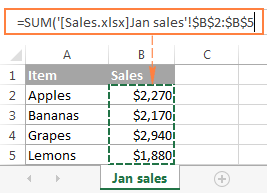Notes:

• When creating a reference to another workbook by selecting the cell(s) in it, Excel always inserts absolute cell references. If you intend to copy the newly created formula to other cells, be sure to remove the dollar sign (\$) from the cell references to turn them into relative or mixed references, depending on your purposes.
• If selecting a cell or range in the referenced workbook does not automatically create a reference in the formula, most likely the two files are open in different instances of Excel. To check this, open Task Manager and see how many Microsoft Excel instances are running. If more than one, expand each instance to view which files are nested there. To fix the issue, close one file (and instance), and then open it again from the other file.

## Reference to a defined name in the same or another workbook

To make an Excel external reference more compact, you can create a defined name in the source sheet, and then refer to that name from another sheet that resides in the same workbook or in a different workbook.

#### Creating a name in Excel

To create a name in Excel, select all the cells you want to include, and then either go to the Formulas tab > Defined names group and click the Define name button, or press Ctrl + F3 and click New.

In the New Name dialog, type any name you want (remember that spaces are not allowed in Excel names), and check if the correct range is displayed in the Refers to field.

For example, this is how we create a name (Jan_sales) for cells B2:B5 in Jan sheet: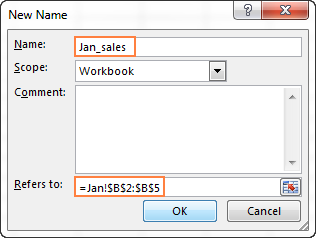Once the name is created, you are free to use it in your external references in Excel. The format of such references is much simpler than the format of an Excel sheet reference and workbook reference discussed earlier, which makes the formulas with name references easier to comprehend.

Note. By default, Excel names are created for the workbook level, please notice the Scope field in the screenshot above. But you can also make a specific worksheet level name by choosing a corresponding sheet from the Scope drop-down list. For Excel references, the scope of a name is very important because it determines the location within which the name is recognized.

It's recommended that you always create workbook-level names (unless you have a specific reason not to), because they significantly simplify creating Excel external references, as illustrated in the following examples.

#### Referencing a name in another sheet in the same workbook

To reference a global workbook-level name in the same workbook, you simply type that name in a function's argument:

=Function(name)

For example, to find the sum of all the cells within the Jan_sales name that we created a moment ago, use the following formula:

`=SUM(Jan_sales)`

To reference a local worksheet-level name in another sheet within the same workbook, you need to precede the name with the sheet name followed by an exclamation mark:

`=Function(Sheet_name!name)`

For example:

`=SUM(Jan!Jan_sales)`

If the sheet names includes spaces or mon-alphabetic chars, remember to enclose it in single quotes, e.g.:

`=SUM('Jan report'!Jan_Sales)`

#### Referencing a name in another workbook

A reference to a workbook-level name in a different workbook consists of the workbook name (including the extension) followed by an exclamation point, and the defined name (named range):

=Function(Workbook_name!name)

For example:

`=SUM(Sales.xlsx!Jan_sales)`

To reference a worksheet-level name in another workbook, the sheet name followed by the exclamation point should be included as well, and the workbook name should be enclosed in square brackets. For example:

`=SUM([Sales.xlsx]Jan!Jan_sales)`

When referencing a named range in a closed workbook, remember to include the full path to your Excel file, for example:

`=SUM('C:\Documents\Sales.xlsx'!Jan_sales)`

#### How to create an Excel name reference

If you have created a handful of different names in your Excel sheets, you don't need to remember all those names by heart. To insert an Excel name reference in a formula, perform the following steps:

1. Select the destination cell, enter the equal sign (=) and start typing your formula or calculation.
2. When it comes to the part where you need to insert an Excel name reference, do one of the following:
• If you are referring to a workbook-level name from another workbook, switch to that workbook. If the name resides in another sheet within the same workbook, skip this step.
• If you are making a reference to a worksheet-level name, navigate to that specific sheet either in the current or different workbook.
3. Press F3 to open the Past Name dialog window, select the name you want to refer to, and click OK.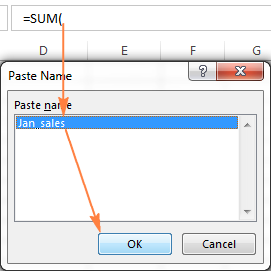4. Finish typing your formula or calculation and press the Enter key.

Now that you know how to create an external reference in Excel, you can take a benefit from this great ability and use data from other worksheets and workbooks in your calculations. I thank you for reading and look forward to seeing you on our blog next week!